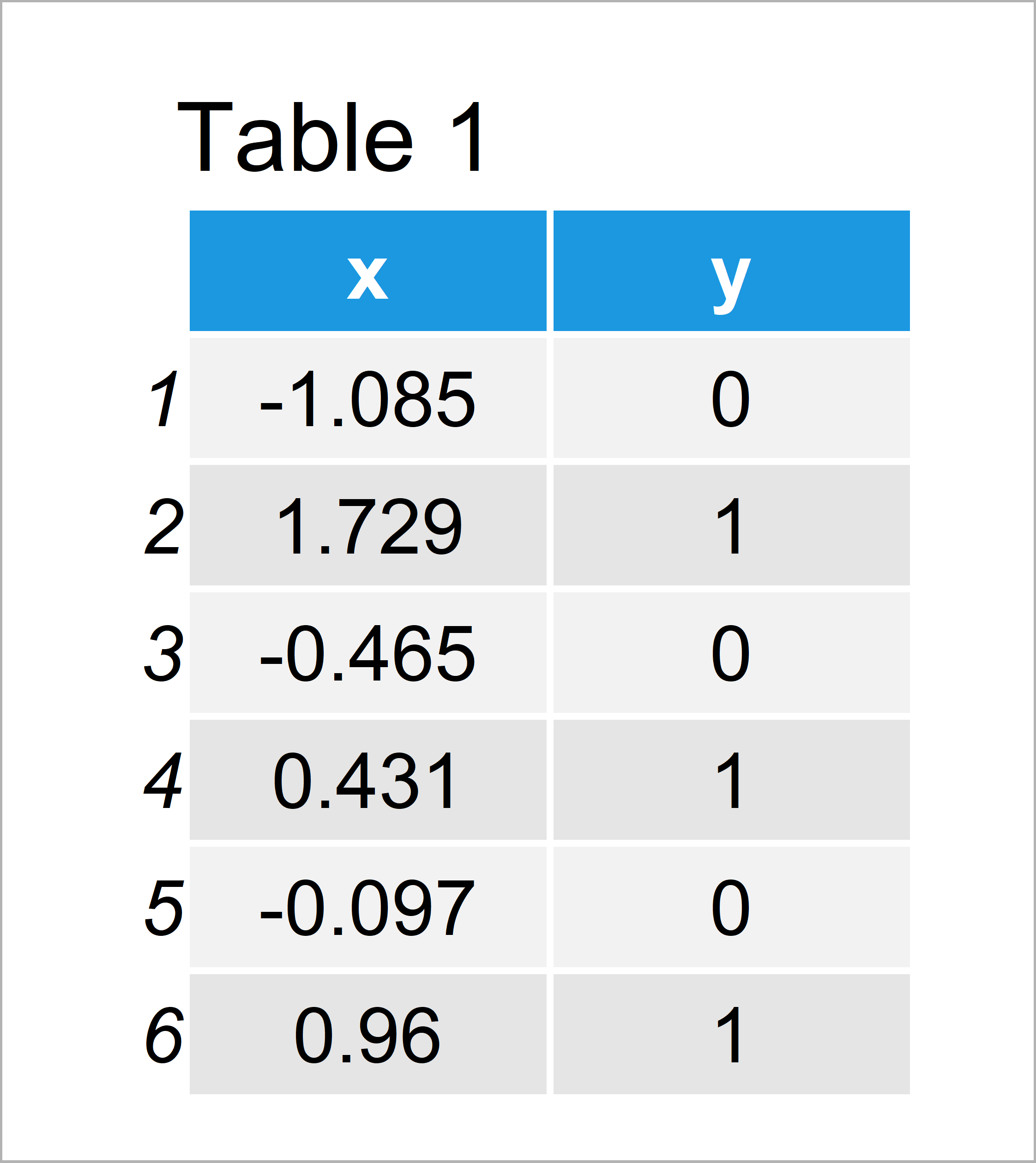# glm.fit Warning Messages in R: algorithm didn’t converge & probabilities 0/1

In this R tutorial you’ll learn how to handle the glm.fit warning: “algorithm did not converge and fitted probabilities numerically 0 or 1”.

The article is structured as follows:

Let’s dive right in.

## Construction of Example Data

The first step is to create some data that we can use in the following examples.

```set.seed(6523987)                            # Create example data
x <- rnorm(100)
y <- rep(1, 100)
y[x < 0] <- 0
data <- data.frame(x, y)Table 1 shows that our example data consists of 100 rows and two columns x and y.

## Example: Reproduce the glm.fit Warning Message – algorithm did not converge fitted probabilities 0 or 1

The following syntax explains why the glm.fit warning: “algorithm did not converge and fitted probabilities numerically 0 or 1” occurs when fitting regression models in the R programming language.

Let’s assume that we want to estimate a binomial model using the glm function. Then we would typically specify our R code as shown below:

```glm(y ~ x, data, family = "binomial")        # Perfect predictions
# Warning messages:
# 1: glm.fit: algorithm did not converge
# 2: glm.fit: fitted probabilities numerically 0 or 1 occurred```

Unfortunately, the glm.fit warning: “algorithm did not converge and fitted probabilities numerically 0 or 1” appears.

The reason for this is that the variable x perfectly predicts the variable y.

You can see that when you go back to the data creation process at the beginning of this tutorial. I have specified that y is always 0 when x is smaller than 0. The model hence perfectly predicts which y values are 0 and which y values are 1.

We can illustrate that by adding some random noise to our data:

```data_new <- data                             # Add randomness to data
data_new\$x <- data_new\$x + rnorm(100)```

Let’s run a glm model with our new data:

```glm(y ~ x, data_new, family = "binomial")    # No warning message
#
# Call:  glm(formula = y ~ x, family = "binomial", data = data_new)
#
# Coefficients:
# (Intercept)            x
#     -0.1049       1.4463
#
# Degrees of Freedom: 99 Total (i.e. Null);  98 Residual
# Null Deviance:	    138
# Residual Deviance: 90.85 	AIC: 94.85```

This time we don’t get a warning message in the RStudio console.

So how can we solve this problem?

This depends heavily on the structure of the specific data set you are using. However, I advise to have a look at the pairwise correlations of the variables in your data to see if some of these correlations are unnaturally high.

It often happens that variables are included to a model by accident. Try to identify these variables and run your model again without these variables until the warning message does not appear anymore.

## Video, Further Resources & Summary

Have a look at the following video of my YouTube channel. I’m showing the R programming syntax of this post in the video:

Furthermore, you may want to have a look at the related tutorials on https://statisticsglobe.com/. I have released numerous tutorials already:

In this R tutorial you have learned how to deal with the glm.fit warning: “algorithm did not converge and fitted probabilities numerically 0 or 1”. In case you have additional questions and/or comments, let me know in the comments.

Subscribe to the Statistics Globe Newsletter